PPWIZARD is a free preprocessor for HTML, REXX, Visual Basic or any text files.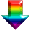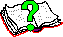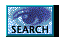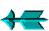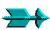\ -> Rexx -> Rexx Expressions

 Rexx Expressions

You can get a rexx manuals (for regina) from http://www.lightlink.com/hessling/, there are HTML and PDF versions available.

The following operators can be used:

• +

• -
Subtracts values.

• *
Multiplies values.

• /
Divides values (5/2 => 2.5).

• %
Divides values, want whole number (5/2 => 2).

• //
Divides values, want remainder (5//2 => 1).

Expressions can also make use of PPWIZARD or standard rexx functions.

Some examples of expressions being used:

```   #evaluate ^^   ^ResultOfExpression = (10 + 8) / 2^        ;;Value 9
#evaluate ^^   ^ResultOfExpression = (_Count - 1) % \$Div^
#evaluate ^^   ^Path               = GetEnv('PATH')^
```

Saturday May 28 2022 at 2:55pm